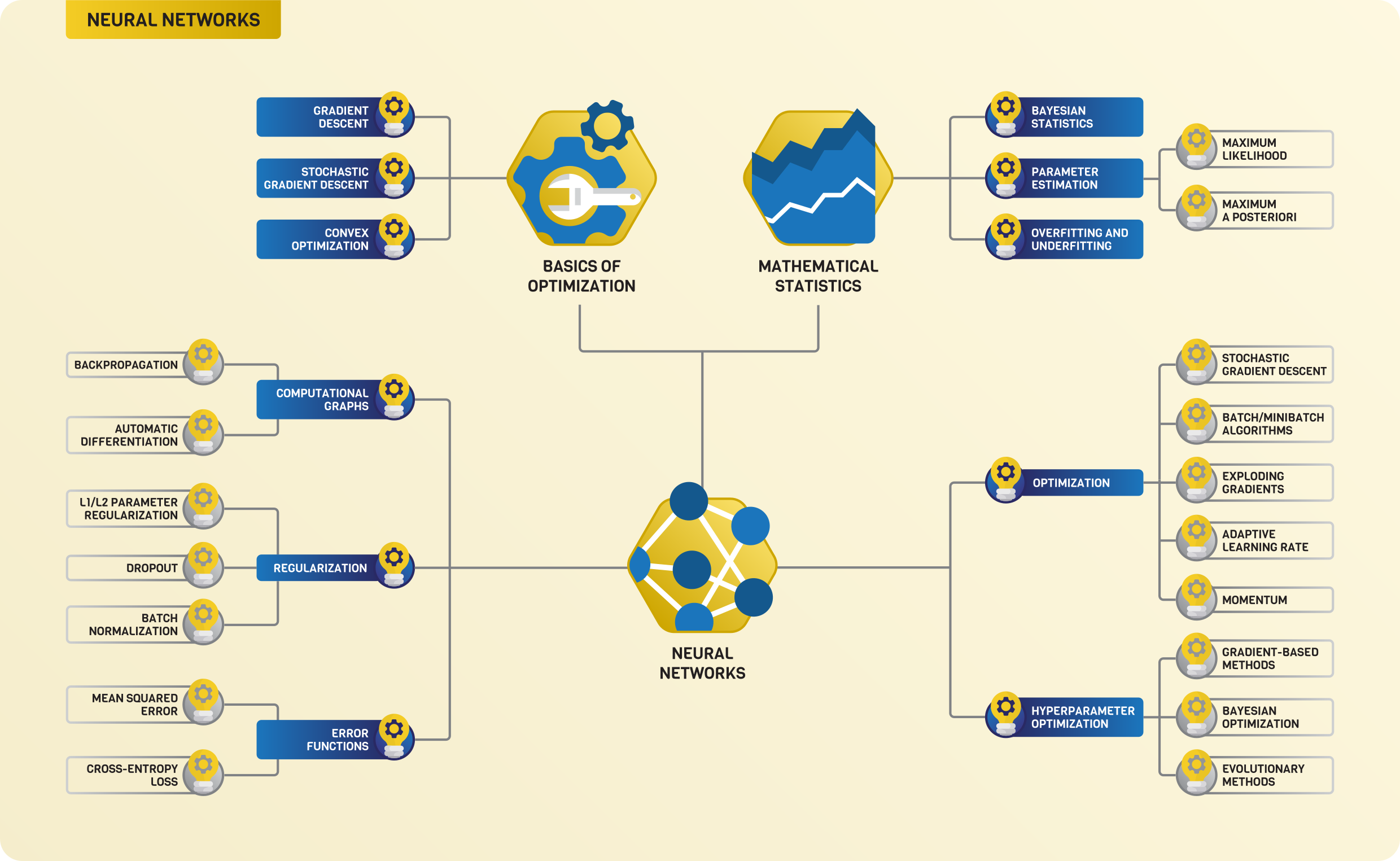# The Roadmap of Mathematics for Machine Learning

Knowing the mathematics behind machine learning algorithms is a superpower. If you have ever built a model for a real-life problem, you probably experienced that familiarity with the details goes a long way if you want to move beyond baseline performance. This is especially true when you want to push the boundaries of state-of-the-art deep learning tools.

However, most of this knowledge is hidden behind layers of advanced mathematics. Understanding methods like stochastic gradient descent might seem challenging since it is built on top of multivariable calculus and probability theory.

If you are a beginner and don't necessarily have formal education in higher mathematics, creating a curriculum for yourself is hard. With proper foundations, though, most ideas can be seen as quite natural. This post aims to present a roadmap of all the mathematics for machine learning, taking you from absolute zero to a deep understanding of how neural networks work.

To keep things simple, the aim is not to cover everything. Instead, we will focus on getting our directions. This way, you will be able to study other topics without difficulties, if need be.

Instead of reading through it in one sitting, I recommend using this article as a reference point throughout your studies. Go deep into a concept that is introduced, then check the roadmap and move on. I firmly believe that this is the best way to study: I will show you the road, but you must walk it.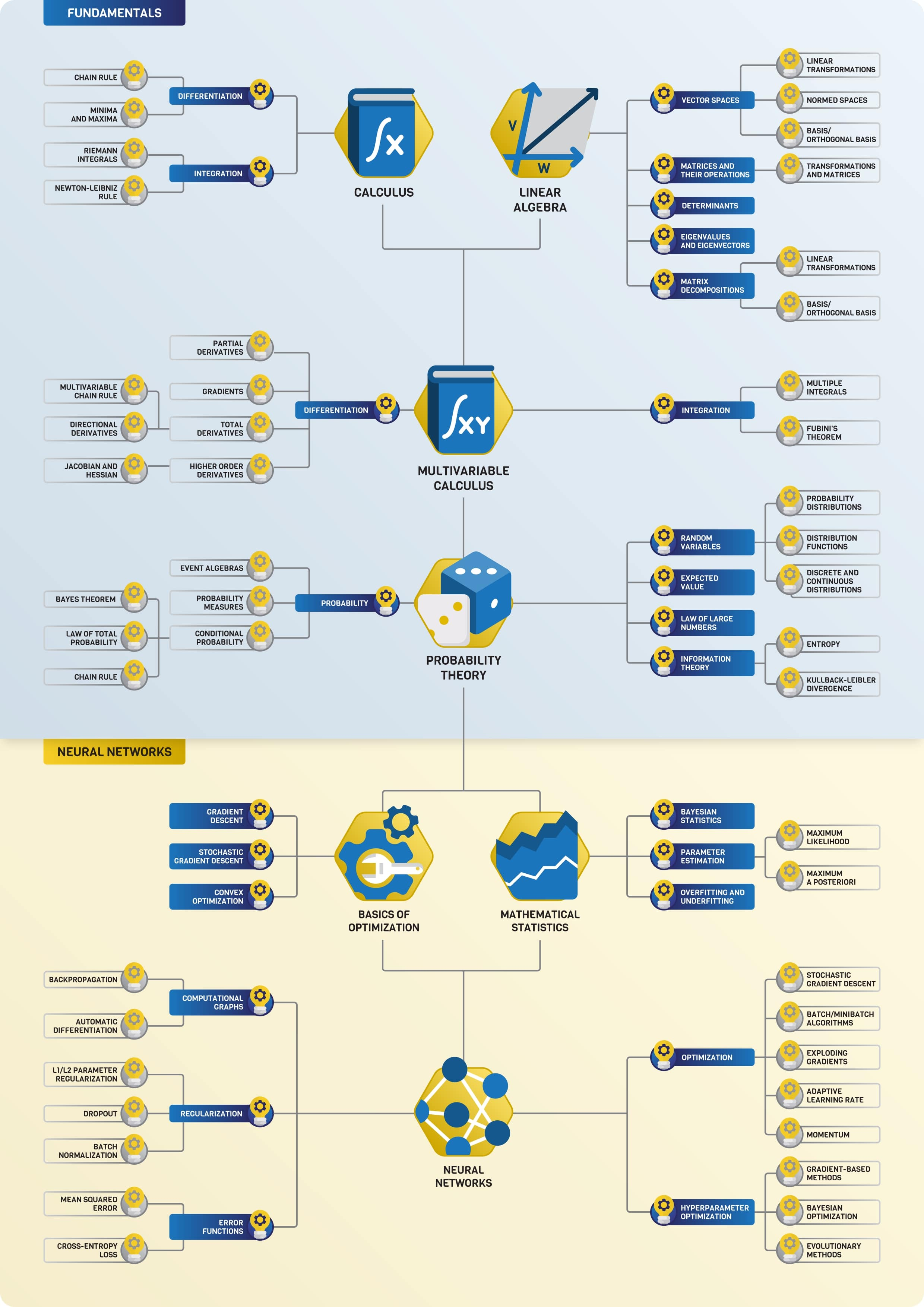Machine learning and deep learning are built upon three pillars: calculus, linear algebra, and probability theory.

## Calculus for machine learning

Calculus is the mathematical study of functions, mainly their differentiation and integration. Essentially, a neural network is a differentiable function, so calculus is a fundamental tool to train neural networks, as we will see.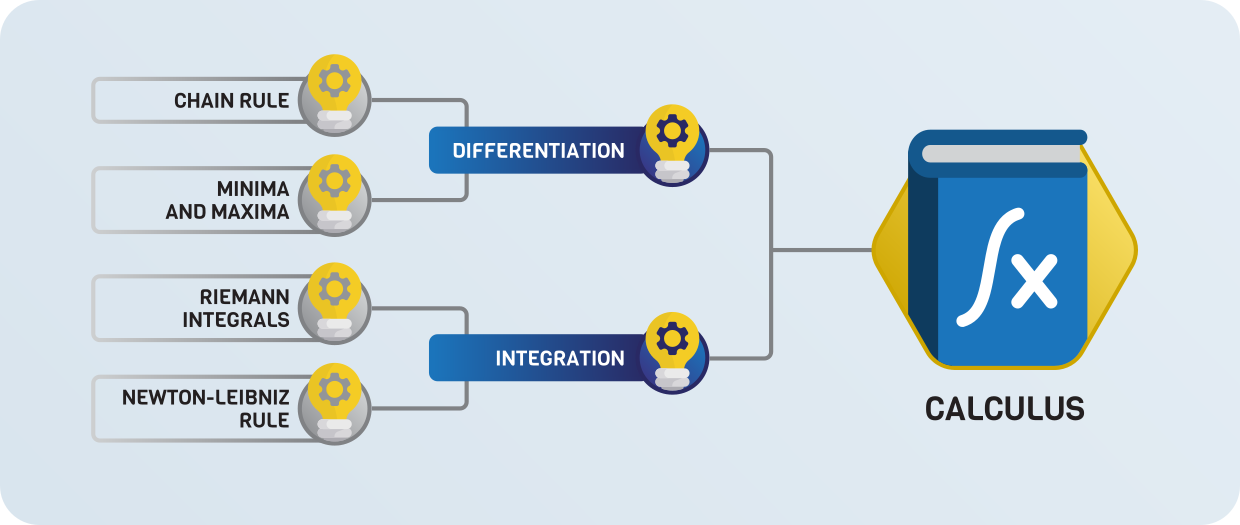### Differentiation

To familiarize yourself with the concepts, you should make things simple and study functions of a single variable for the first time. By definition, the derivative of a function is defined by

$f^\prime(x) = \lim_{h \to 0} \frac{f(x + h) - f(x)}{h},$

where the ratio for a given $\textstyle h$ is the slope of the line between the points $(x, f(x))$ and $(x+h, f(x+h))$.

This is essentially the slope of the tangent line at the point $\textstyle x$ in the limit. The figure below illustrates the concept.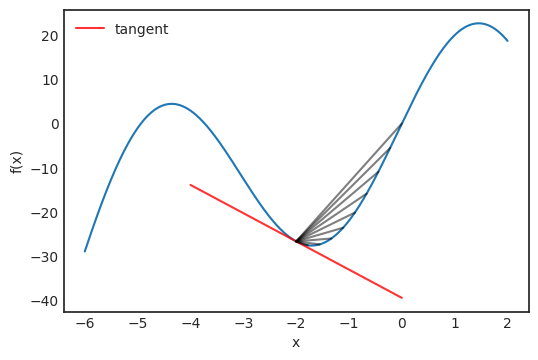We can use differentiation to optimize functions: the derivative is zero at local maxima or minima. (However, this is not true in the other direction; see $f(x) = x^3$ at $\textstyle 0$.) Points where the derivative is zero are called critical points. Whether a critical point $x_0$ is minima or maxima can be decided by looking at the second derivative:

$f^{\prime\prime}(x_0) = \begin{cases} > 0: & x_0 \text{ is a local minima}, \\ < 0: & x_0 \text{ is a local maxima}, \\ = 0: & \text{undetermined}. \end{cases}$

This characterization of extremal points with the second derivative is the very foundation of optimization in deep learning, allowing down the line to train neural networks by gradient descent.

There are several essential rules regarding differentiation, but probably the most important is the so-called chain rule:

$\big( f(g(x))\big)^\prime = f^\prime(g(x)) g^\prime(x)$

that tells us how to calculate the derivative of composed functions.

### Integration

Integration is often called the inverse of differentiation. This is true because

\begin{align*} F(x) &= f(x_0) \int_{x_0}^{x} f(x) dx \\ F^\prime(x) &= f(x), \end{align*}

which holds for any integrable function $f(x)$. The integral of a function can also be thought of as the signed area under the curve. For instance,

$\int_{-\pi}^{\pi} \sin x dx = 0$

because when the function is negative, the area there also has a negative sign.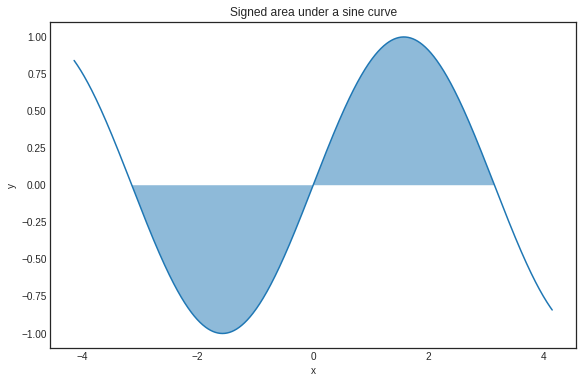Integration itself plays a role in understanding the concept of expected value. For instance, quantities like entropy and Kullback-Leibler divergence are defined in terms of integrals.

### Further study

I would recommend the Single Variable Calculus course from MIT (In general, online courses from MIT are always excellent learning resources.) If you are more of a book person, there are many textbooks available. The Calculus book by Gilbert Strang accompanying the previously mentioned course is again a great resource, completely free of charge.

## Linear algebra for machine learning

As I mentioned, neural networks are essentially functions, which are trained using the tools of calculus. However, they are described with linear algebraic concepts like matrix multiplication. Hence, linear algebra plays a crucial role in deep learning.### Vector spaces

To have a good understanding of linear algebra, I would suggest starting with vector spaces. It is better if we talk about a special case first. You can think of each point in the plane as a tuple $x = (x_1, x_2) \in \mathbb{R}^2$. These are essentially vectors pointing from zero to $(x_1, x_2)$. You can add these vectors together and multiply them with scalars:

\begin{align*} (x_1, x_2) + (y_1, y_2) &= (x_1 + y_1, x_2 + y_2), \\ a(x_1, x_2) &= (ax_1, ax_2), \quad a \in \mathbb{R}. \end{align*}

This is the prototypical model of a vector space. In general, a set of vectors $\textstyle V$ is a vector space over the real numbers if you can add vectors together and multiply a vector with a real number, such that the following properties hold:

\begin{align*} (1) \quad & x + y = y + x \text{ for all } x, y \in V, \\ (2) \quad & x + (y + z) = (x + y) + z \text{ for all } x, y, z \in V, \\ (3) \quad & \text{there is an element } 0 \in V \text{ such that for all } x \in V, \text{ we have } v + 0 = v, \\ (4) \quad & \text{for every } x \in V, \text{ there is an } y \in V \text{ such that } x + y = 0, \\ (5) \quad & a(bx) = (ab)x \text{ for all } a, b \in \mathbb{R}, x \in V, \\ (6) \quad & 1x = x \text{ for all } x \in V, \\ (7) \quad & (a + b)x = ax + bx \text{ for all } a, b \in \mathbb{R}, x \in V, \\ (8) \quad & a(x + y) = ax + ay \text{ for all } a \in \mathbb{R}, x, y \in V. \end{align*}

Don't panic! I know that this looks very scary (at least it looked that to me when I was a freshman student with a math major), but it isn't. These properties guarantee that vectors can be added together and scaled just as you would expect. When thinking about vector spaces, it helps if you mentally model them as the Euclidean plane.

### Normed spaces

If you feel like you have a good understanding of vector spaces, the next step would be to understand how to measure a vector's magnitude. By default, a vector space in itself gives no tools for this. How would you do this on the plane? You have probably learned that there, we have

$|x| = \sqrt{(x_1)^2 + (x_2)^2}.$

This is a special case of a norm. In general, a vector space $\textstyle V$ is normed if there is a function $| \cdot |: V \to [0, \infty)$ called norm such that

\begin{align*} (1) \quad & |x| \geq 0, \quad x \in V, \\ (2) \quad & |x| = 0 \text{ if and only if } x = 0, \\ (3) \quad & |ax| = |a| |x|, \quad a \in \mathbb{R}, x \in V, \\ (4) \quad & |x+y| \leq |x| + |y|, \quad x, y \in V. \end{align*}

Again, this might be scary, but this is a simple and essential concept. There are a bunch of norms out there, but the most important is the p-norm family

$|x|_p = \bigg( \sum_{i=1}^{n}(x_i)^p \bigg)^{1/p}, \quad p \in [1, \infty), x \in \mathbb{R}^n$

(with $p = 2$ we obtain the special case mentioned above) and the supremum norm

$|x|_\infty = \sup { x_1, \dots, x_n}, \quad x \in \mathbb{R}^n.$

Sometimes, like for $p = 2$, the norm comes from a so-called inner product, which is a bilinear function

$\langle \cdot, \cdot \rangle: V \times V \to [0, \infty),$

such that

\begin{align*} (1) \quad & \langle x, y \rangle = \langle y, x \rangle, \\ (2) \quad & \langle ax + by, z \rangle = a \langle x, z \rangle + b \langle y, z \rangle, \\ (3) \quad & \langle x, x \rangle \geq 0, \\ (4) \quad & \langle x, x \rangle = 0 \iff x = 0. \end{align*}

A vector space with an inner product is called inner product space. An example is the classical Euclidean product

$\langle x, y \rangle = x_1 y_1 + x_2 y_2.$

Every inner product can be turned into a norm by

$|x| = \sqrt{\langle x, x \rangle}.$

When the inner product for two vectors is zero, we say that the vectors are orthogonal to each other. (Try to come up with some concrete examples on the plane to understand the concept deeper!)

### Basis and orthogonal/orthonormal basis

Although vector spaces are infinite (in our case), you can find a finite set of vectors that can be used to express all vectors in the space. For example, on the plane, we have

$x = (x_1, x_2) = x_1 e_1 + x_2 e_2,$

where

\begin{align*} e_1 &= (1, 0), \\ e_2 &= (0, 1). \end{align*}

This is a special case of basis and orthonormal basis. In general, a basis is a minimal set of vectors $b_1, b_2, \dots, b_n \in V$ such that their linear combinations span the vector space:

$V = \bigg\{ \sum_{i=1}^{n} \alpha_i b_i: a_i \in \mathbb{R} \bigg\}.$

A basis always exists for any vector space. (It may not be a finite set, but that shouldn't concern us now.) Without a doubt, a basis simplifies things greatly when talking about linear spaces.

When the vectors in a basis are orthogonal to each other, we call it an orthogonal basis. If each basis vector's norm is $\textstyle 1$ for an orthogonal basis, we say it is orthonormal.

### Linear transformations

The key objects related to vector spaces are linear transformations. If you have seen a neural network before, you know that one of the fundamental building blocks are layers of the form $f(x) = \sigma(Ax + b)$ where $\textstyle A$ is a matrix, $\textstyle b$ and $\textstyle x$ are vectors, and $\textstyle \sigma$ is the Sigmoid function. (Or any activation function, really.) Well, the part $Ax$ is a linear transformation. In general, the function $L: V \to W$ is a linear transformation between vector spaces $\textstyle V$ and $\textstyle W$ if

$L(ax + by) = aL(x) + bL(y)$

holds for all $x, y \in V$ and all real number $\textstyle a$. Deep neural networks can be (mostly) described with a successive application of linear transformations, with interspersed nonlinear functions (such as activations).

To give a concrete example, rotations around the origin in the plane are linear transformations. Undoubtedly, the most crucial fact about linear transformations is that they can be represented with matrices, as you'll see next in your studies.

### Matrices and their operations

If linear transformations are clear, you can turn to the study of matrices. (Linear algebra courses often start with matrices, but I would recommend it this way for reasons to be explained later.)

The most important operation for matrices is the matrix product. In general, if $\textstyle A$ and $\textstyle B$ are matrices defined by

\begin{align*} A &= (a_{i, j})_{i, j = 1}^{n, m} \in \mathbb{R}^{n \times m}, \\ B &= (b_{i, j})_{i, j = 1}^{m, l} \in \mathbb{R}^{m \times l}. \end{align*}

then their product can be obtained by

$AB = \Big( \sum_{k=1} a_{i, k} b_{k, j} \Big)_{i,j = 1}^{n, l}.$

This might seem difficult to comprehend, but it is pretty straightforward. Take a look at the figure below, demonstrating how to calculate the element in the 2nd row, 1 st column of the product.The reason why matrix multiplication is defined the way it is because matrices represent linear transformations between vector spaces. Matrix multiplication is the composition of linear transformations.

### Determinants

In my opinion, determinants are hands down one of the most challenging concepts to grasp in linear algebra. Depending on your learning resource, it is usually defined by either a recursive definition or a sum that iterates through all permutations. None of them are tractable without significant experience in mathematics.

To understand this concept, watch this video. Trust me, it is magic.

To summarize, the determinant of a matrix describes how the volume of an object scales under the corresponding linear transformation. If the transformation changes orientations, the sign of the determinant is negative. You will eventually need to understand how to calculate the determinant, but I wouldn't worry about it now.

### Eigenvalues, eigenvectors, and matrix decompositions

A standard first linear algebra course usually ends with eigenvalues/eigenvectors and some special matrix decompositions like the Singular Value Decomposition.

Let's suppose that we have a matrix $\textstyle A$. The number $\textstyle \lambda$ is an eigenvalue of $\textstyle A$ if there is a vector $\textstyle x$ (called eigenvector) such that

$Ax = \lambda x$

holds. In other words, the linear transformation represented by $\textstyle A$ is a scaling by $\textstyle \lambda$ for the vector $\textstyle x$. This concept plays an essential role in linear algebra. (And practically in every field which uses linear algebra extensively.)

At this point, you are ready to familiarize yourself with a few matrix decompositions. If you think about it for a second, what type of matrices are the best from a computational perspective? Diagonal matrices! If a linear transformation has a diagonal matrix, it is trivial to compute its value on an arbitrary vector.

$\begin{bmatrix} \lambda_1 & 0 & \dots & 0 \\ 0 & \lambda_2 & \dots & 0 \\ \vdots & \vdots & \ddots & \vdots \\ 0 & 0 & \dots & \lambda_n \end{bmatrix} \begin{bmatrix} x_1 \\ x_2 \\ \vdots \\ x_n \end{bmatrix} = \begin{bmatrix} \lambda_1 x_1 \\ \lambda_2 x_2 \\ \vdots \\ \lambda_n x_n \end{bmatrix}$

Most special forms aim to decompose a matrix $\textstyle A$ to a product of matrices, where preferably at least one is diagonal. Singular Value Decomposition, or SVD in short, the most famous one, states that there are special matrices $U, V$, and a diagonal matrix $\Sigma$ such that

$A = U \Sigma V$

holds. ($\textstyle U$ and $\textstyle V$ are so-called unitary matrices, which I don't define here, suffice to know that it is a special family of matrices.)

SVD is also used to perform Principal Component Analysis, one of the simplest and most well-known dimensionality reduction methods.

### Further study

Linear algebra can be taught in many ways. The path I outlined here was inspired by the textbook Linear Algebra Done Right) by Sheldon Axler. For an online lecture, I would recommend the Linear Algebra course from MIT OpenCourseWare, an excellent resource.

## Multivariable calculus for machine learning

This is the part where linear algebra and calculus come together, laying the foundations for the primary tool of deep learning to train neural networks: gradient descent. Mathematically speaking, a neural network is simply a function of multiple variables. (Although, the number of variables can be in the millions.)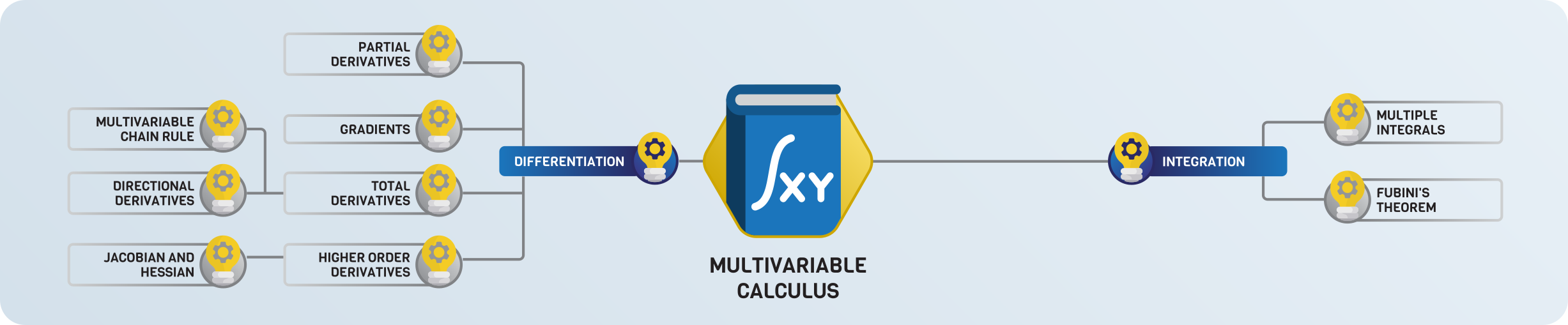Similar to univariable calculus, the two main topics here are differentiation and integration. Let's suppose that we have a function $f: \mathbb{R}^n \to \mathbb{R}$, mapping vectors to real numbers. In two dimensions (that is, for $n = 2$), you can imagine its plot as a surface. (Since humans don't see higher than three dimensions, it is hard to visualize functions with more than two real variables.)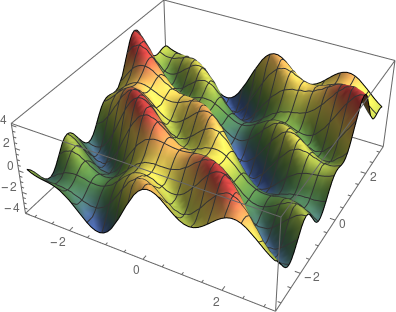### Differentiation in multiple variables

In a single variable, the derivative was the slope of the tangent line. How would you define tangents here? A point on the surface has several tangents, not just one. However, there are two special tangents: one is parallel to the $x-z$ plane, while the other is to the $y-z$ plane. Their slope is determined by the partial derivatives, defined by

\begin{align*} \frac{\partial f}{\partial x} &= \lim_{h \to 0} \frac{f(x + h, y) - f(x, y)}{h}, \\ \frac{\partial f}{\partial y} &= \lim_{h \to 0} \frac{f(x, y + h) - f(x, y)}{h}. \\ \end{align*}

That is, you take the derivative of the functions obtained by fixing all but one variable. (The formal definition is identical for more than three variables, just more complicated notation.) Tangents in these special directions span the tangent plane.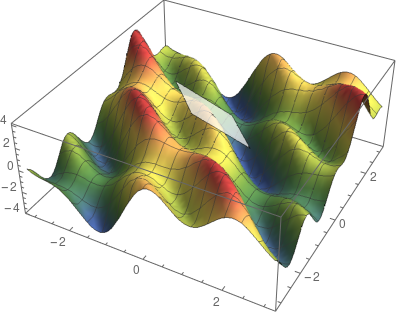There is another special direction: the gradient, which is the vector defined by

$\nabla f(x_1, x_2, \dots, x_n) = \bigg( \frac{\partial f}{\partial x_1}, \frac{\partial f}{\partial x_2}, \dots, \frac{\partial f}{\partial x_n} \bigg).$

The gradient always points to the direction of the largest increase! So, if you would take a tiny step in this direction, your elevation would be the maximal among all the other paths you could have chosen. This is the basic idea of gradient descent, which is an algorithm to maximize functions. Its steps are the following.

1. Calculate the gradient in the point $x_0$, where you currently are.
2. Take a small step in the direction of the gradient to arrive at the point $x_1$. (The step size is called the learning rate.)
3. Go back to Step 1. and repeat the process until convergence.

Of course, there are several flaws in this basic algorithm, which was improved several times over the years. Modern gradient descent-based optimizers employ many tricks like adaptive step size, momentum, and other methods, which we will not detail here.

Calculating the gradient in practice is difficult. Functions are often described by the composition of other functions, for instance, the familiar linear layer

$f(x) = \sigma(Ax + b),$

where $\textstyle A$ is a matrix, $\textstyle b$ and $\textstyle x$ are vectors, and $\sigma$ is the sigmoid function. (Of course, there can be are other activations, but we shall stick to this for simplicity.) How would you calculate this gradient? At this point, it is unclear how to define the gradient for vector-vector functions such as this, so let's discuss! A function $g(x): \mathbb{R}^n \to \mathbb{R}^m$ can always be written in terms of vector-scalar functions like

$g(x) = \begin{bmatrix} g_1(x) \\ g_2(x) \\ \vdots \\ g_m(x) \end{bmatrix}, \quad g_i : \mathbb{R}^n \to \mathbb{R}.$

The gradient of $\textstyle g$ is defined by the matrix whose $\textstyle k$-th row is the $\textstyle k$-th component's gradient. That is,

$\Delta g(x) = \Big( \frac{\partial}{\partial x_i} g_j(x) \Big)_{i, j = 1}^{n, m}.$

This matrix is called the total derivative of $\textstyle g$.

In our example $f(x) = \sigma(Ax + b)$, things become a bit more complicated because it is the composition of two functions:

$l: \mathbb{R}^n \to \mathbb{R}^m, \quad x \mapsto Ax + b$

and

$\sigma: \mathbb{R}^m \to \mathbb{R}^m,$

defined by applying the univariate sigmoid componentwise. We can decompose the function $\textstyle l$ further to $\textstyle m$ functions mapping from the $\textstyle n$-dimensional vector space to the space of real numbers:

$l(x) = Ax + b = \begin{bmatrix} l_1(x) \\ l_2(x) \\ \vdots \\ l_m(x) \end{bmatrix},$

where

$l_i(x) = \sum_{j=1}^{n} a_{i, j} x_j + b_i.$

If you calculate the total derivative, you'll see that

$\Delta f(x) = \Delta \sigma(l(x)) \cdot \Delta l(x).$

This is the chain rule for multivariate functions in its full generality. Without it, there would be no easy way to calculate the gradient of a neural network, which is ultimately a composition of many functions.

### Higher-order derivatives

Similar to the univariate case, the gradient and the derivatives play a role in determining whether a given point in space is local minima or maxima. (Or neither.) To provide a concrete example, training a neural network is equivalent to minimizing the loss function on the parameters' training data. It is all about finding the optimal parameter configuration w for which the minimum is attained:

$\min_{w} l(N(x_{\text{train}}, w)),$

where

$N: \mathbb{R}^n \to \mathbb{R}^m, \quad l: \mathbb{R}^m \to \mathbb{R}$

are the neural network and the loss function, respectively. For a general differentiable vector-scalar function of say n variables, there are $n^2$ second derivatives, forming the Hessian matrix

$Hf(x) = \begin{bmatrix} \frac{\partial^2 f}{\partial x_1^2} & \frac{\partial^2 f}{\partial x_1 \partial x_2} & \dots & \frac{\partial^2 f}{\partial x_1 \partial x_n} \\ \frac{\partial^2 f}{\partial x_2 \partial x_1} & \frac{\partial^2 f}{\partial x_2^2} & \dots & \frac{\partial^2 f}{\partial x_2 \partial x_n} \\ \vdots & \vdots & \ddots & \vdots \\ \frac{\partial^2 f}{\partial x_n \partial x_1} & \frac{\partial^2 f}{\partial x_n \partial x_2} & \dots & \frac{\partial^2 f}{\partial x_n^2} \\ \end{bmatrix}.$

In multiple variables, the determinant of the Hessian takes the role of the second derivative. Similarly, we can use it to tell whether a critical point (that is, where all of the derivatives are zero) is minima, maxima, or just a saddle point.

### Further study

There are a lot of awesome online courses on multivariable calculus. I have two specific recommendations:

These contain much more than what is needed for machine learning, so there is ample opportunity to go deeper into the mathematics of multivariable functions. (Which is good news, since higher-dimensional functions are beautiful!)

Now we are ready to take on the final subject: probability theory!

## Probability theory for machine learning

Probability theory is the mathematically rigorous study of chance, fundamental to all fields of science. In machine learning, probability theory is essential to make predictive decisions under uncertainty.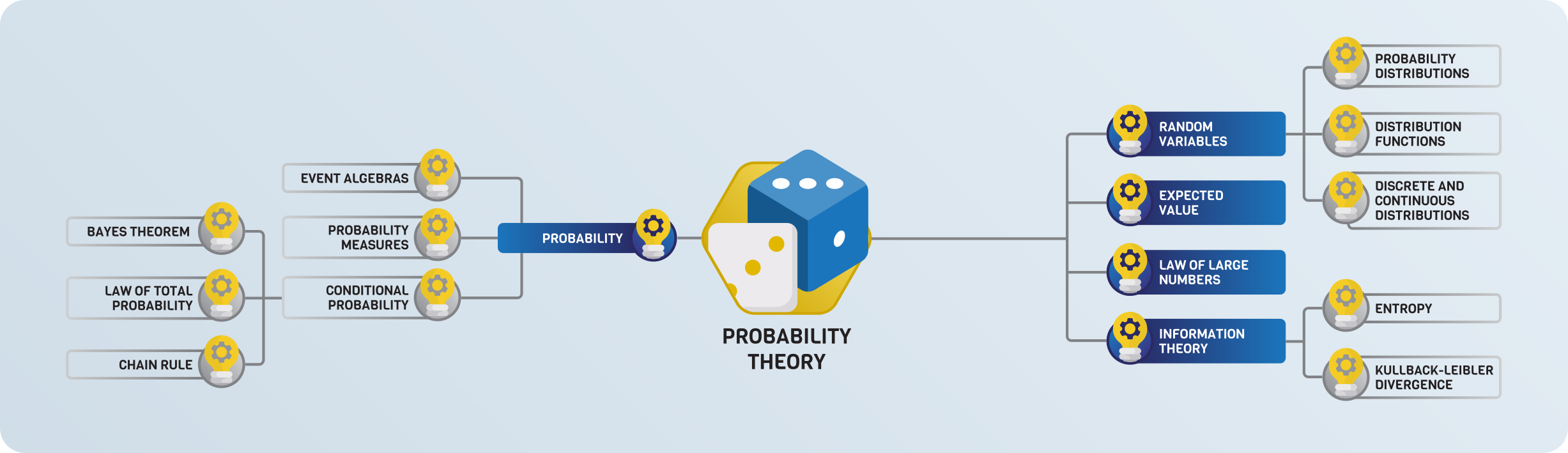Let's start our journey with the very basics! Setting exact definitions aside, let's ponder a bit about what probability represents. Let's say I toss a coin, with a $50\%$ chance (or $0.5$ probability) of it being heads. After repeating the experiment 10 times, how many heads did I get?

If you have answered 5, you are wrong. (And even if you are right, you are most likely right for the wrong reasons.) Heads being 0.5 probability doesn't guarantee that every second throw is heads. Instead, a given probability means that if you repeat the experiment $\textstyle n$ times where $\textstyle n$ is a very large number, the number of heads will be very close to $n/2$.

Besides the basics, there are some advanced things you need to understand, first and foremost, expected value and entropy. Let's start

### Expected value

Suppose that you play a game with your friend. You toss a classical six-sided dice, and if the outcome is 1 or 2, you win 300 bucks. Otherwise, you lose 200. What are your average earnings per round if you play this game long enough? Should you be even playing this game?

Well, you win 100 bucks with probability $1/3$, and you lose 200 with probability $2/3$. That is, if $\textstyle X$ is the random variable encoding the result of the dice throw, then

\begin{align*} E[X] &= 300 P(X \in \{1, 2\}) - 200 P(X \in \{3, 4, 5, 6\}) \\ &= 300 \frac{1}{3} - 200 \frac{2}{3} \\ &= - \frac{100}{3}. \end{align*}

This is the expected value, that is, the average amount of money you will receive per round in the long run. Since this is negative, you will lose money, so you should never play this game.

Generally speaking, the expected value is defined by

$E[X] = \sum_{i=1}^{\infty} x_i P(X = x_i), \quad X \in \{x_1, x_2, \dots \}$

for discrete random variables and

$E[X] = \int_{-\infty}^{\infty} x f_X(x) dx$

for real-valued continuous random variables.

In machine learning, loss functions for training neural networks are expected values in one way or another.

### Law of large numbers

People often falsely attribute certain phenomena to the law of large numbers. For instance, gamblers on a losing streak believe that they should soon win because of the law of large numbers. This is wrong. Let's see what this is really!

Suppose that $X_1, X_2, \dots$, are random variables representing the independent repetitions of the same experiment. (Say, rolling a dice or tossing a coin.)

Essentially, the law of large numbers states that

$\lim_{n \to \infty} \frac{1}{n} \sum_{i=1}^{n} X_i = E[X],$

that is, the average of the outcomes in the long run is equal to the expected value.

An interpretation is that if a random event is repeated enough times, individual results might not matter. So, if you are playing in a casino with a game that has negative expected value (as they all do), it doesn't matter that you win occasionally. The law of large numbers implies that you will lose money.

To get a little bit ahead, LLN is going to be essential for stochastic gradient descent.

### Information theory

Let's play a game. I have thought of a number between 1 and 1024, and you have to guess it. You can ask questions, but your goal is to use as few questions as possible. How much do you need?

If you play it smart, you will perform a binary search with your questions. First, you may ask: is the number between 1 and 512? With this, you have cut the search space in half. Using this strategy, you can figure out the answer in $\log_2 1024 = 10$ questions.

But what if I didn't use the uniform distribution when picking the number? For instance, I could have used a Poisson distribution. (Illustrated below, source: Wikipedia.)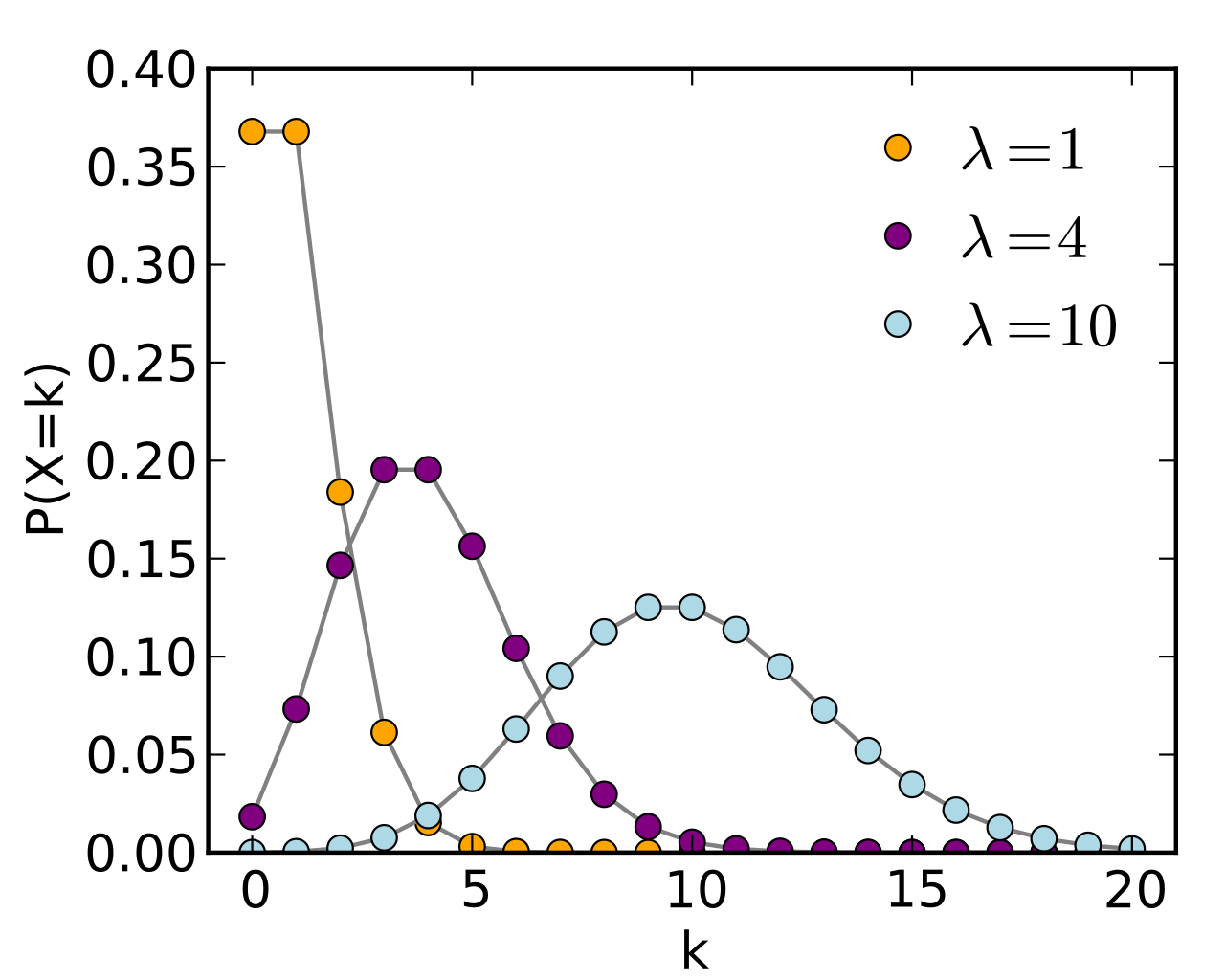Here, you would probably need fewer questions because you know that the distribution tends to concentrate around specific points. (Which depends on the parameter.)

In the extreme case, when the distribution is concentrated on a single number, you need zero questions to guess it correctly. Generally, the number of questions depends on the information carried by the distribution. The uniform distribution contains the least amount of information, while singular ones are pure information.

Entropy is a way to quantify this. It is defined by

$H[X] = - \sum_{i=1}^{\infty} P(x_i) \log P(x_i)$

for discrete random variables and

$H[X] = \int_{-\infty}^{\infty} f(x) \log f(x) dx$

for continuous, real-valued ones. (The logarithm base is usually 2, $\textstyle e$, or 10, but it doesn't really matter.)

If you have ever worked with classification models before, you probably encountered the cross-entropy loss, defined by

$L[X] = - \sum_{i=1}^{\infty} P(x_i) \log \hat{P}(x_i),$

where $\textstyle P$ is the ground truth (a distribution concentrated to a single class), while the hatted version represents the class predictions. This measures how much "information" the predictions have compared to the ground truth. When the predictions match, the cross-entropy loss is zero.

Another frequently used quantity is the Kullback-Leibler divergence, defined by

$D_{KL}(P || Q) = - \sum_{i=1}^{\infty} P(x_i) \log \frac{Q(x_i)}{P(x_i)},$

where $\textstyle P$ and $\textstyle Q$ are two probability distributions. This is essentially cross-entropy minus the entropy, which can be thought of as quantifying how different the two distributions are. This quantity is useful, for instance, when training generative adversarial networks. Minimizing the Kullback-Leibler divergence guarantees that the two distributions are similar.

### Further study

Here, I would recommend two books for you:

These are the two fundamental textbooks, and they teach you much more than probability theory. Both of them go way beyond the basics, but the corresponding chapters provide an excellent introduction.

## Beyond the mathematical foundations

With this, we reviewed the all the mathematics for understanding neural networks. Now, you are ready for the fun part: machine learning! To understand how neural networks work, you still have to learn some optimization and mathematical statistics. These subjects build upon the foundations we set. I won't go into details since this is beyond the scope of the article, but I have prepared a study roadmap to guide you.